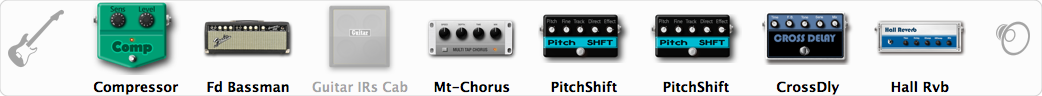# Is this love?

Discussion in 'ToneLib-GFX presets' started by schllr, Aug 10, 2019.

1. Is this love?

Preset name: Chorused clean tone

Effects chain:Effect: "Compressor" (Dynamics / Filter), active - "yes"
"Sense" = 31
"Level" = 29

Effect: "Fd Bassman" (Amp simulators), active - "yes"
"Gain" = 31
"Bass" = 63
"Middle" = 97
"Treble" = 63
"Presence" = 54
"Master" = 58
"Output" = 85
"Level (dB)" = 0

Effect: "Guitar IRs Cab" (Cabinets), active - "no"
"Model" = Fender Twin Rev (2x12")
"Mic Position" = Edge
"Mic Distance" = Near
"Low Cut (Hz)" = 60
"Hi Cut (kHz)" = 20.0
"Mix" = 100
"Level (dB)" = 0

Effect: "Mt-Chorus" (Modulation / Sfx), active - "yes"
"Speed" = 5.3
"Depth" = 47
"Time" = 2.5
"Mix" = 48

Effect: "PitchShift" (Modulation / Sfx), active - "yes"
"Pitch" = 0
"Fine" = 8
"Tracking" = 89
"Direct" = 100
"Effect" = 67

Effect: "PitchShift" (Modulation / Sfx), active - "yes"
"Pitch" = 0
"Fine" = -9
"Tracking" = 85
"Direct" = 100
"Effect" = 52

Effect: "CrossDly" (Delay), active - "yes"
"Time" = 36
"Feedback" = 0
"Tone" = 58
"Sens" = 0
"Mix" = 100

Effect: "Hall Rvb" (Reverb), active - "yes"
"Time" = 9.4
"PreDelay" = 61
"LoDamp" = 49
"HiDamp" = 17
"Mix" = 44

Note: You will need to download and install the ToneLib-GFX software to use the preset.

File size:
725 bytes
Views:
14,688